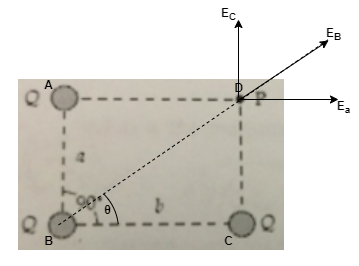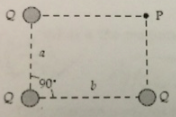# Problem: If Q = 16 nC, a = 3.0 m, and b. 4.0 m, what is the magnitude of the electric field at point?

###### FREE Expert Solution

The electric field is expressed as:

$\overline{){\mathbf{E}}{\mathbf{=}}\frac{\mathbf{k}\mathbf{q}}{{\mathbf{r}}^{\mathbf{2}}}}$

The magnitude of the electric field is given by:

$\overline{){\mathbf{E}}{\mathbf{=}}\sqrt{{{\mathbf{E}}_{\mathbf{x}}}^{\mathbf{2}}\mathbf{+}{{\mathbf{E}}_{\mathbf{y}}}^{\mathbf{2}}}}$

Let's visualize our problem in the figure below:89% (225 ratings)###### Problem Details

If Q = 16 nC, a = 3.0 m, and b. 4.0 m, what is the magnitude of the electric field at point?